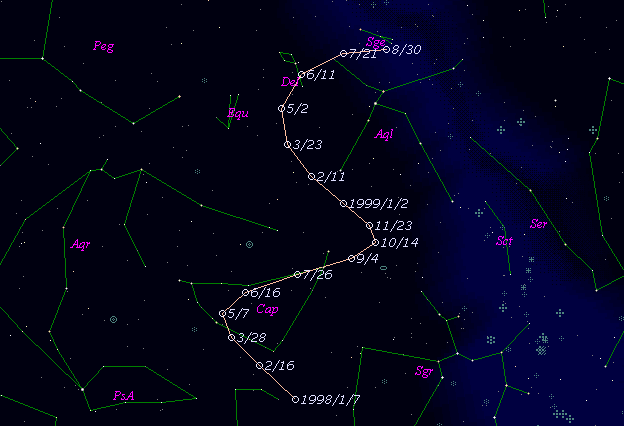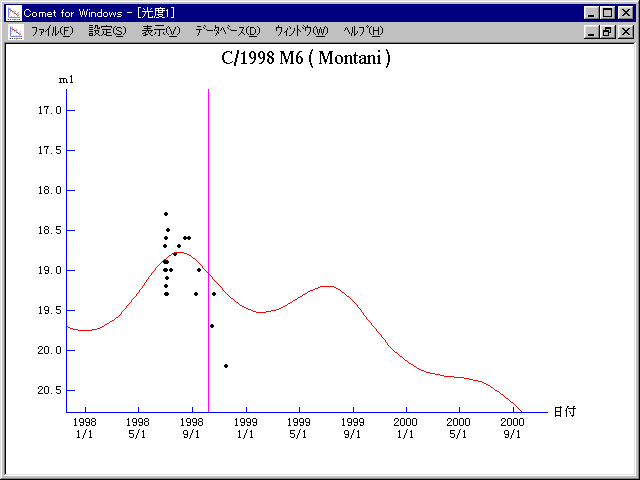# \$B%b%s%?!<%KWB@1(B

C/1998 M6 ( Montani )###\$B%W%m%U%#!<%k(B

 \$BH/8+F|(B 1998\$BG/(B6\$B7n(B30\$BF|(B \$BH/8+8wEY(B 18.9\$BEy(B \$BH/8+ J. Montani

###\$B50F;MWAG(B

```   The following improved orbital elements by Kenji Muraoka, are
from 86 observations  1998 June 30  to  Nov. 16, perturbations
by 9 Planets, Moon and 5 minor planets were taken into account.
The mean residual is +/- 0.66 arc seconds.

Epoch  =  1998 Sept. 24.0  TT      JDT = 2451080.5
T  =  1998 Oct.   6.56562      +/- 0.10252 (m.e.) TT
Peri. =    9.14640                +/- 0.00737
Node  =  306.60361                +/- 0.00264   (2000.0)
Incl. =   91.54037                +/- 0.00608
q  =    5.9786745              +/- 0.0001491 AU
e  =    0.9989914              +/- 0.0001179
1/a  =   +0.0001687              +/- 0.0000197 1/AU
```

###\$B@1?^(B###\$B8wEYJQ2=(B

```        m1 = 7.5 + 5 log\$B&\$(B + 10.0 log r
```##### \$B50F;MWAG\$OB<2,7r<#;a\$N7W;;\$K\$h\$k\$b\$N\$G\$9!#(B \$B@1?^\$O(B StellaNavigator Ver.2.0 for Windows (\$B%"%9%H%m%"!<%D(B \$BJTCx(B / \$B%"%9%-!<=PHG6I4)(B) \$B\$G:n@.\$7\$?\$b\$N\$G\$9!#(B \$B8wEY%0%i%U\$O(BComet for Windows\$B\$G:n@.\$7\$?\$b\$N\$G\$9!#(B